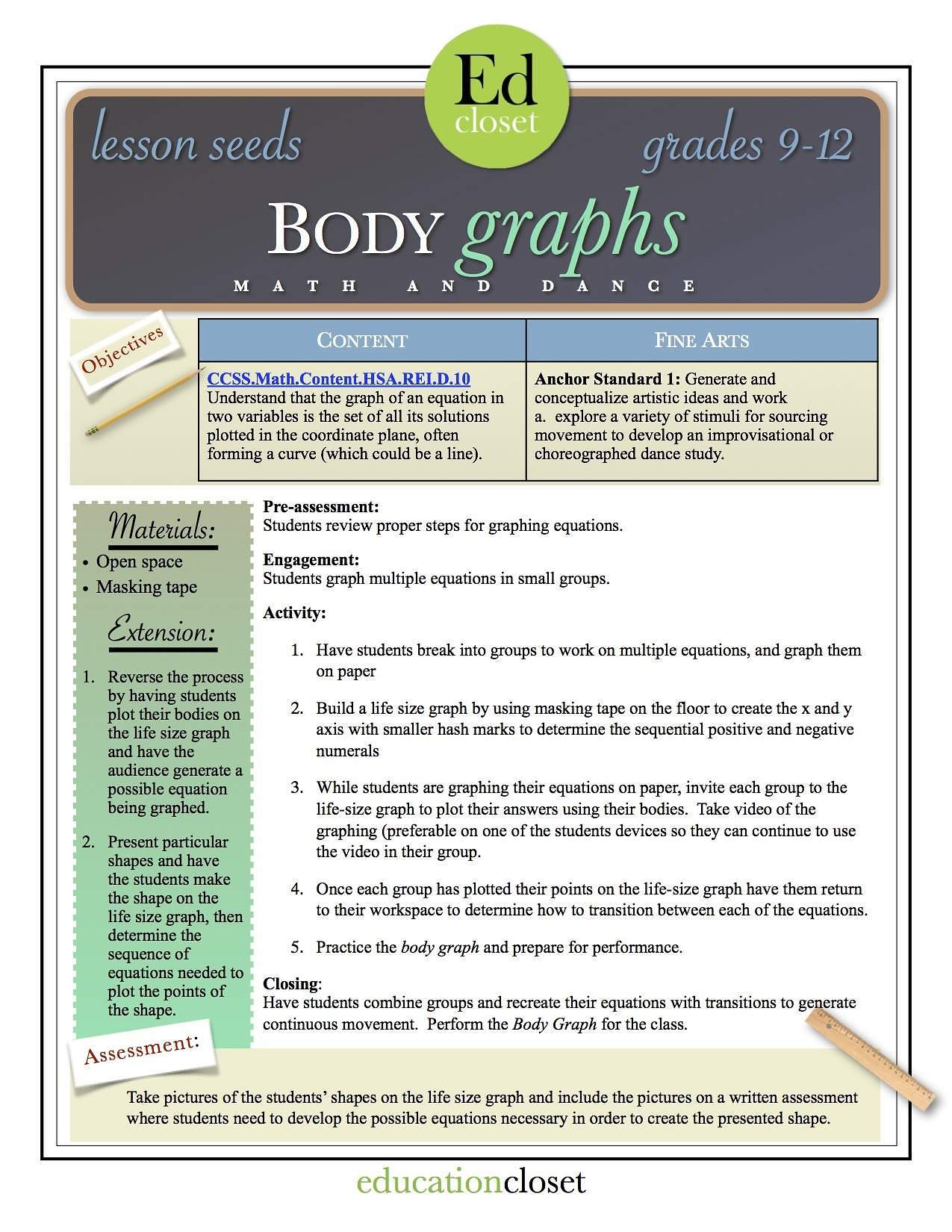Typhani Harris | August 2015

# Studio STEAM-up: Math and Dance

Welcome to the fifth and final installment of the Studio STEAM-up Series!

Each week we have explored arts integrated lessons that connect dance to the STEAM subjects.  This final week is Math and Dance!  This was by far the most difficult for me to conceptualize.  I’ll be honest, being an artist, the logical progression of math and dance is quite confusing.  I guess it’s that whole right brain vs left brain phenomenon.  However, there are multiple opportunities to invite movement into the math and dance class.

### StandardsCommon Core MathNCCAS Dance

#### Lesson Seeds

Any opportunity for students to move around the space creates a strong mind to body connection allowing for a faster recall.  The Common Core math and dance standards for high school are separated into six categories: number and quantity, algebra, functions, modeling, geometry, and statistics & probability.

#### Number and Quantity Under number and quantity is quantification.  Something that stood out to me was

” Quantification is also important for companies, which must conceptualize relevant attributes and create or choose suitable measures for them”  (www.corestandards.org).

So why not have students quantify the necessary space needed for a suitable dance studio?  Assuming that the studio is being constructed for the students in class, quantify the space needed for each individual kinesphere, and build the studio measurements based on the needs of the class.

#### Algebra Rearranging equations to solve for different parts is essential in algebra.  Common Core standards state:

“Rearrange formulas to highlight a quantity of interest, using the same reasoning as in solving equations.” (www.corestandards.org)

Create specific movement for each quantity and have students rearrange the movement phrase based on the quantity of interest.  For example: let’s look at Ohm’s law of V = IR.  Give each quantity a specific movement, V – I – and R.  Then have students rearrange the movement phrase to solve for I or to solve for R.  Not only will this allow you to assess for students mastery of rearranging equations but also will give students a kinesthetic reminder of the formula which in turn will create greater recall.  Don’t forget to create movement for the functions adding, subtracting, multiplying, and dividing!

#### Functions Under function is the expectation of building functions, one standard is:

“Combine standard function types using arithmetic operations” (www.corestandards.org)

So, why not take that to the studio?   For example, build a function that models the temperature of a cooling body.  Then test the function by dancing.  Raise the body temperature through movement and use the function to determine the rate of the cooling body.  Then check for correctness and understanding.

#### Modeling Modeling is a great opportunity to integrate dance and dance related topics.

“The basic modeling cycle is summarized in the diagram. It involves (1) identifying variables in the situation and selecting those that represent essential features, (2) formulating a model by creating and selecting geometric, graphical, tabular, algebraic, or statistical representations that describe relationships between the variables, (3) analyzing and performing operations on these relationships to draw conclusions, (4) interpreting the results of the mathematics in terms of the original situation, (5) validating the conclusions by comparing them with the situation, and then either improving the model or, if it is acceptable, (6) reporting on the conclusions and the reasoning behind them” (www.corestandards.org)

### Have students model the cost of designing and building a studio.

Or, the monthly overhead and profit of running a studio.  How much would they charge each student in order to make a profit.  Or for producing a concert, how much will it take for the venue, costumes, programs, tickets, etc?  How much would you have to charge in order to make a profit on the performance?  Place these hypotheticals in real-life.  Have students research actual venues, contact printing companies, and check how much electricity will be used.

#### Geometry

A large expectation in geometry is understanding theorems and subsequently proving the theorems.  This provides a wonderful opportuntiy to integrate dance.  Create movement patterns for each of the theorems.  This process alone will help students to retain their theorem knowledge, but you can go even further by having students use their geometric proofs as a composition map.  Organize the theorem phrases into a dance and perform the proofs for the class.   Have students evaluate their proofs for correctness.

#### Statistics & Probability

Making inferences and determining probability is a foundation of this section.  Why not take the phrases made for theorems or algebraic functions and determine the quantity of possible arrangements of the phrases.  Or determine the statistical probability of the Pythagorean theorem phrase being the first phrase performed.  Have student view a traditional jazz class and collect data on various skills.  For example: successful completion of multiple pirouettès at the beginning of class versus the end of class, or the height of a battement before stretching versus after stretching, then graph the findings noting trends in the data.

## Lesson Plan

While the lesson activities will allow you to assess students understanding of graphing equations, the extension activities will really push your students to higher levels of thinking and understanding.  Consider using the extention as a final assessment of student knowledge.Body Graph Lesson

There are so many opportunities to connect math and dance   If you have a math lesson you would like to integrate with dance, or if you try one of these here please drop me a comment below or email me at [email protected], I would love to hear about it!

Piquès & Pirouettès
-Typh

Next Week: Studio STEAM-up Recap!